## Module 10: Quadratic Equations and Functions

10.3 – applications of quadratic functions, learning objectives, objects in free fall.

• Determining the width of a border## Finding the maximum and minimum values of a quadratic function

(10.3.1) – solve application problems involving quadratic functions.

Quadratic equations are widely used in science, business, and engineering. Quadratic equations are commonly used in situations where two things are multiplied together and they both depend on the same variable. For example, when working with area, if both dimensions are written in terms of the same variable, you use a quadratic equation. Because the quantity of a product sold often depends on the price, you sometimes use a quadratic equation to represent revenue as a product of the price and the quantity sold. Quadratic equations are also used when gravity is involved, such as the path of a ball or the shape of cables in a suspension bridge.

A very common and easy-to-understand application is the height of a ball thrown at the ground off a building. Because gravity will make the ball speed up as it falls, a quadratic equation can be used to estimate its height any time before it hits the ground. Note: The equation isn’t completely accurate, because friction from the air will slow the ball down a little. For our purposes, this is close enough.

A ball is thrown off a building from 200 feet above the ground. Its starting velocity (also called initial velocity ) is $−10$ feet per second. (The negative value means it’s heading toward the ground.)

The equation $h=-16t^{2}-10t+200$ can be used to model the height of the ball after $t$ seconds. About how long does it take for the ball to hit the ground?

When the ball hits the ground, the height is 0. Substitute 0 for $h$.

$\begin{array}{c}h=-16t^{2}-10t+200\\0=-16t^{2}-10t+200\\-16t^{2}-10t+200=0\end{array}$

This equation is difficult to solve by factoring or by completing the square, so solve it by applying the Quadratic Formula, $x=\frac{-b\pm \sqrt{{{b}^{2}}-4ac}}{2a}$. In this case, the variable is $t$ rather than $x$. $a=−16,b=−10$, and $c=200$.

$\displaystyle t=\frac{-(-10)\pm \sqrt{{{(-10)}^{2}}-4(-16)(200)}}{2(-16)}$

Simplify. Be very careful with the signs.

$\large \begin{array}{l}t=\frac{10\pm \sqrt{100+12800}}{-32}\\\,\,=\frac{10\pm \sqrt{12900}}{-32}\end{array}$

Use a calculator to find both roots.

$t$ is approximately $−3.86$ or $3.24$.

Consider the roots logically. One solution, $−3.86$, cannot be the time because it is a negative number. The other solution, $3.24$ seconds, must be when the ball hits the ground.

The ball hits the ground approximately $3.24$ seconds after being thrown.

In the next video we show another example of how the quadratic equation can be used to find the time it takes for an object in free fall to hit the ground.

Here are some more similar objects in free fall examples.

## Example: Applying the Vertex and $x$ -Intercepts of a Parabola

A ball is thrown upward from the top of a 40 foot high building at a speed of 80 feet per second. The ball’s height above ground can be modeled by the equation $H\left(t\right)=-16{t}^{2}+80t+40$.

a. When does the ball reach the maximum height?

b. What is the maximum height of the ball?

c. When does the ball hit the ground?

$\large \begin{array}{c} h=-\frac{80}{2\left(-16\right)} \text{ }=\frac{80}{32}\hfill \\ \text{ }=\frac{5}{2}\hfill \\ \text{ }=2.5\hfill \end{array}$

The ball reaches a maximum height after 2.5 seconds.

b. To find the maximum height, find the y  coordinate of the vertex of the parabola.

$\large \begin{array}{c}k=H\left(-\frac{b}{2a}\right)\hfill \\ \text{ }=H\left(2.5\right)\hfill \\ \text{ }=-16{\left(2.5\right)}^{2}+80\left(2.5\right)+40\hfill \\ \text{ }=140\hfill \end{array}$

The ball reaches a maximum height of 140 feet.

c. To find when the ball hits the ground, we need to determine when the height is zero, $H\left(t\right)=0$.

$\large \begin{array}{c} t=\frac{-80\pm \sqrt{{80}^{2}-4\left(-16\right)\left(40\right)}}{2\left(-16\right)}\hfill \\ \text{ }=\frac{-80\pm \sqrt{8960}}{-32}\hfill \end{array}$

Because the square root does not simplify nicely, we can use a calculator to approximate the values of the solutions.

$\large \begin{array}{c}t=\frac{-80-\sqrt{8960}}{-32}\approx 5.458\hfill & \text{or}\hfill & t=\frac{-80+\sqrt{8960}}{-32}\approx -0.458\hfill \end{array}$

The second answer is outside the reasonable domain of our model, so we conclude the ball will hit the ground after about 5.458 seconds.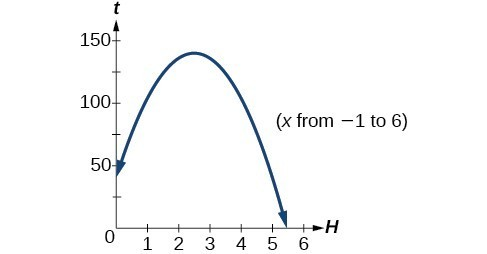A rock is thrown upward from the top of a 112-foot high cliff overlooking the ocean at a speed of 96 feet per second. The rock’s height above ocean can be modeled by the equation $H\left(t\right)=-16{t}^{2}+96t+112$.

a. When does the rock reach the maximum height?

b. What is the maximum height of the rock?

c. When does the rock hit the ocean?

a. 3 seconds

b. 256 feet

c. 7 seconds

## Applications of quadratic functions: determining the width of a border

The area problem below does not look like it includes a Quadratic Formula of any type, and the problem seems to be something you have solved many times before by simply multiplying. But in order to solve it, you will need to use a quadratic equation.

Bob made a quilt that is 4 ft $\times$ 5 ft. He has 10 sq. ft. of fabric he can use to add a border around the quilt. How wide should he make the border to use all the fabric? (The border must be the same width on all four sides.)

Sketch the problem. Since you don’t know the width of the border, you will let the variable $x$ represent the width.

In the diagram, the original quilt is indicated by the red rectangle. The border is the area between the red and blue lines.

Since each side of the original 4 by 5 quilt has the border of width x added, the length of the quilt with the border will be $5+2x$, and the width will be $4+2x$.

(Both dimensions are written in terms of the same variable, and you will multiply them to get an area! This is where you might start to think that a quadratic equation might be used to solve this problem.)

You are only interested in the area of the border strips. Write an expression for the area of the border.

Area of border = Area of the blue rectangle minus the area of the red rectangle

Area of border$=\left(4+2x\right)\left(5+2x\right)–\left(4\right)\left(5\right)$

There are 10 sq ft of fabric for the border, so set the area of border to be 10.

$10=\left(4+2x\right)\left(5+2x\right)–20$

Multiply $\left(4+2x\right)\left(5+2x\right)$.

$10=20+8x+10x+4x^{2}–20$

$10=18x+4x^{2}$

Subtract 10 from both sides so that you have a quadratic equation in standard form and can apply the Quadratic Formula to find the roots of the equation.

$\begin{array}{c}0=18x+4x^{2}-10\\\\\text{or}\\\\4x^{2}-10\\\\2\left(2x^{2}+9x-5\right)=0\end{array}$

Factor out the greatest common factor, 2, so that you can work with the simpler equivalent equation, $2x^{2}+9x–5=0$.

$\large \begin{array}{r}2\left(2x^{2}+9x-5\right)=0\\\\\frac{2\left(2x^{2}+9x-5\right)}{2}=\frac{0}{2}\\\\2x^{2}+9x-5=0\end{array}$

Use the Quadratic Formula. In this case, $a=2,b=9$, and $c=−5$.

$\large \begin{array}{l}x=\frac{-b\pm \sqrt{{{b}^{2}}-4ac}}{2a}\\\\x=\frac{-9\pm \sqrt{{{9}^{2}}-4(2)(-5)}}{2(2)}\end{array}$

$\displaystyle x=\frac{-9\pm \sqrt{121}}{4}=\frac{-9\pm 11}{4}$

Find the solutions, making sure that the $\pm$ is evaluated for both values.

$\large \begin{array}{c}x=\frac{-9+11}{4}=\frac{2}{4}=\frac{1}{2}=0.5\\\\\text{or}\\\\x=\frac{-9-11}{4}=\frac{-20}{4}=-5\end{array}$

Ignore the solution $x=−5$, since the width could not be negative.

The width of the border should be 0.5 ft.

Here is a video which gives another example of using the quadratic formula for a geometry problem involving the border around a quilt.

There are many real-world scenarios that involve finding the maximum or minimum value of a quadratic function, such as applications involving area and revenue.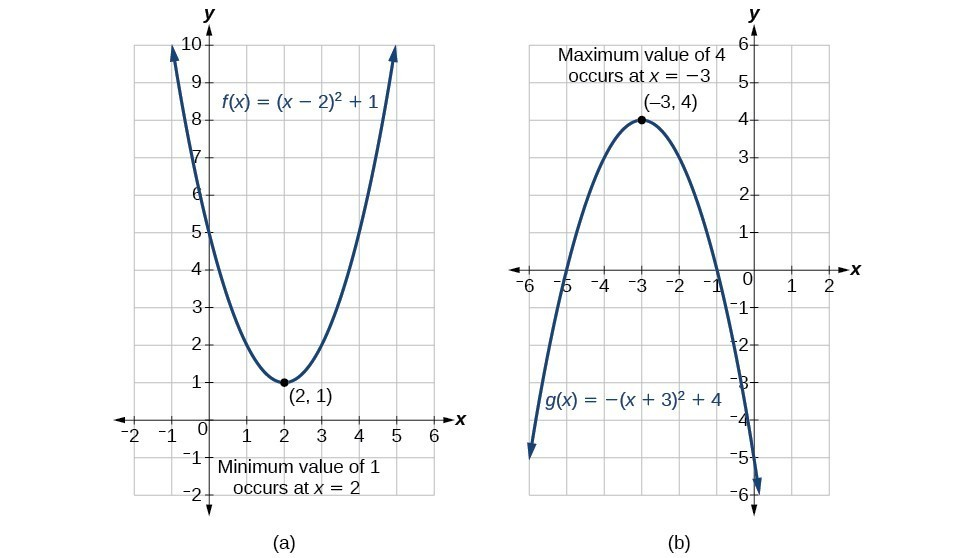Find two numbers $x$ and $y$ whose difference is 100 and whose product is a minimum.

We are trying to find the minimum of the product $P=xy$ of two numbers, such that their difference is 100: $y-x=100$. First, we rewrite one variable in terms of the other:

$y-x=100 \rightarrow y=100+x$

Next, we plug in the above relationship between the variables into the first equation:

$P=xy=x(100+x) = 100x+x^2 = x^2+100x$

As a result, we get a quadratic function $P(x)=x^2+100x$. The graph of this quadratic function opens upwards, and its vertex is the minimum, So if we find the vertex of this parabola, we will find the minimum product. The vertex is:

$\displaystyle \displaystyle \left(-\frac{b}{2a}, P\left(-\frac{b}{2a}\right)\right) = \left(-\frac{(100)}{2(1)}, P\left(-\frac{(100)}{2(1)}\right)\right) = (-50,-2,500)$

Thus the minimum of the parabola occurs at $x=-50$, and is $-2,500$. So one of the numbers is $x=-50$, the other we obtain by plugging in $x=-50$ in to $y-x=100$:

$\begin{array}{cc}y-(-50)&=&100 \\ y+50 &=& 100 \\ y &=& 50\end{array}$

$x=-50$ and $y=50$

## Example: Finding the Maximum Value of a Quadratic Function

A backyard farmer wants to enclose a rectangular space for a new garden within her fenced backyard. She has purchased 80 feet of wire fencing to enclose three sides, and she will use a section of the backyard fence as the fourth side.

• Find a formula for the area enclosed by the fence if the sides of fencing perpendicular to the existing fence have length $L$.
• What dimensions should she make her garden to maximize the enclosed area?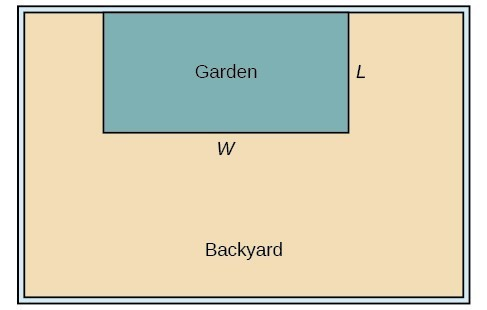Let’s use a diagram such as the one above to record the given information. It is also helpful to introduce a temporary variable, W , to represent the width of the garden and the length of the fence section parallel to the backyard fence.

1)  We know we have only 80 feet of fence available, and $L+W+L=80$, or more simply, $2L+W=80$. This allows us to represent the width, $W$, in terms of $L$.

$W=80 - 2L$

Now we are ready to write an equation for the area the fence encloses. We know the area of a rectangle is length multiplied by width, so

$\begin{array}{l}\text{ }A&=&LW=L\left(80 - 2L\right)\hfill \\ A\left(L\right)&=&80L - 2{L}^{2}\hfill \end{array}$

This formula represents the area of the fence in terms of the variable length $L$. The function, written in general form, is

$A\left(L\right)=-2{L}^{2}+80L$.

2) The quadratic has a negative leading coefficient, so the graph will open downward, and the vertex will be the maximum value for the area. In finding the vertex, we must be careful because the equation is not written in standard polynomial form with decreasing powers. This is why we rewrote the function in general form above. Since $a$ is the coefficient of the squared term, $a=-2,b=80$, and $c=0$.

To find the vertex:

$\large \begin{array}{l}h=-\frac{80}{2\left(-2\right)}\hfill & \hfill & \hfill & \hfill & k=A\left(20\right)\hfill \\ \text{ }=20\hfill & \hfill & \text{and}\hfill & \hfill & \text{ }=80\left(20\right)-2{\left(20\right)}^{2}\hfill \\ \hfill & \hfill & \hfill & \hfill & \text{ }=800\hfill \end{array}$

The maximum value of the function is an area of 800 square feet, which occurs when $L=20$ feet. When the shorter sides are 20 feet, there is 40 feet of fencing left for the longer side. To maximize the area, she should enclose the garden so the two shorter sides have length 20 feet and the longer side parallel to the existing fence has length 40 feet.

## Analysis of the Solution

This problem also could be solved by graphing the quadratic function. We can see where the maximum area occurs on a graph of the quadratic function below.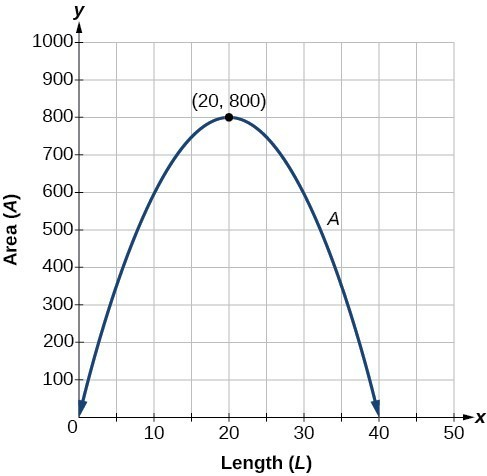## How To: Given an application involving revenue, use a quadratic equation to find the maximum.

• Write a quadratic equation for revenue.
• Find the vertex of the quadratic equation.
• Determine the $y$-value of the vertex.

## Example: Finding Maximum Revenue

The unit price of an item affects its supply and demand. That is, if the unit price goes up, the demand for the item will usually decrease. For example, a local newspaper currently has 84,000 subscribers at a quarterly charge of $30. Market research has suggested that if the owners raise the price to$32, they would lose 5,000 subscribers. Assuming that subscriptions are linearly related to the price, what price should the newspaper charge for a quarterly subscription to maximize their revenue?

Revenue is the amount of money a company brings in. In this case, the revenue can be found by multiplying the price per subscription times the number of subscribers, or quantity. We can introduce variables, $p$ for price per subscription and $Q$ for quantity, giving us the equation $\text{Revenue}=pQ$.

Because the number of subscribers changes with the price, we need to find a relationship between the variables. We know that currently $p=30$ and $Q=84,000$. We also know that if the price rises to $32, the newspaper would lose 5,000 subscribers, giving a second pair of values, $p=32$ and $Q=79,000$. From this we can find a linear equation relating the two quantities. The slope will be $\large \begin{array}{c}m=\frac{79,000 - 84,000}{32 - 30}\hfill \\ \text{ }=\frac{-5,000}{2}\hfill \\ \text{ }=-2,500\hfill \end{array}$ This tells us the paper will lose 2,500 subscribers for each dollar they raise the price. We can then solve for the y -intercept. $\begin{array}{c}\text{ }Q=-2500p+b\hfill & \text{Substitute in the point }Q=84,000\text{ and }p=30\hfill \\ 84,000=-2500\left(30\right)+b\hfill & \text{Solve for }b\hfill \\ \text{ }b=159,000\hfill & \hfill \end{array}$ This gives us the linear equation $Q=-2,500p+159,000$ relating cost and subscribers. We now return to our revenue equation. $\begin{array}{c}\text{Revenue}=pQ\hfill \\ \text{Revenue}=p\left(-2,500p+159,000\right)\hfill \\ \text{Revenue}=-2,500{p}^{2}+159,000p\hfill \end{array}$ We now have a quadratic function for revenue as a function of the subscription charge. To find the price that will maximize revenue for the newspaper, we can find the vertex. $\large \begin{array}{c}h=-\frac{159,000}{2\left(-2,500\right)}\hfill \\ \text{ }=31.8\hfill \end{array}$ The model tells us that the maximum revenue will occur if the newspaper charges$31.80 for a subscription. To find what the maximum revenue is, we evaluate the revenue function.

$\begin{array}{c}\text{maximum revenue}&=&-2,500{\left(31.8\right)}^{2}+159,000\left(31.8\right)\hfill \\ \text{ }&=&2,528,100\hfill \end{array}$

This could also be solved by graphing the quadratic. We can see the maximum revenue on a graph of the quadratic function.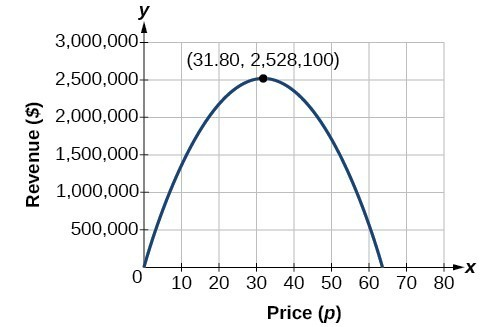A coordinate grid has been superimposed over the quadratic path of a basketball in the picture below. Find an equation for the path of the ball. Does the shooter make the basket?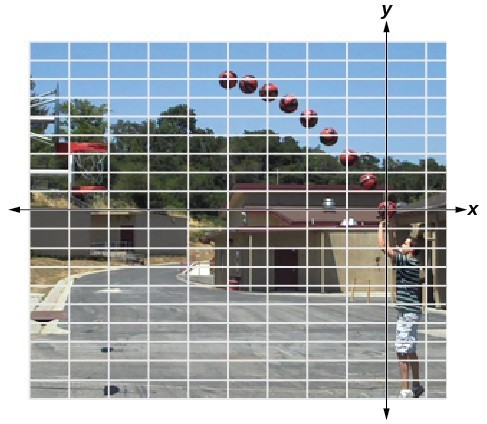(credit: modification of work by Dan Meyer)

The path passes through the origin and has vertex at $\left(-4,\text{ }7\right)$, so $\left(h\right)x=-\frac{7}{16}{\left(x+4\right)}^{2}+7$. To make the shot, $h\left(-7.5\right)$ would need to be about 4 but $h\left(-7.5\right)\approx 1.64$; he doesn’t make it.

• Quadratic Formula Application - Time for an Object to Hit the Ground. Authored by : James Sousa (Mathispower4u.com) for Lumen Learning. Located at : https://youtu.be/RcVeuJhcuL0 . License : CC BY: Attribution
• Quadratic Formula Application - Determine the Width of a Border. Authored by : James Sousa (Mathispower4u.com) for Lumen Learning. Located at : https://youtu.be/Zxe-SdwutxA . License : CC BY: Attribution
• Pre-Algebra Topics
• Algebra Topics
• Algebra Calculator
• Algebra Cheat Sheet
• Algebra Practice Test
• Algebra Formulas
• Want to Build Your Own Website?

Yes, I know it's tough. You've finally mastered factoring and using the quadratic formula and now you are asked to solve more problems!

Except these are even more tough. Now you have to figure out what the problem even means before trying to solve it. I completely understand and here's where I am going to try to help!

There are many types of problems that can easily be solved using your knowledge of quadratic equations. You may come across problems that deal with money and predicted incomes (financial) or problems that deal with physics such as projectiles. You may also come across construction type problems that deal with area or geometry problems that deal with right triangles.

Lucky for you, you can solve the quadratic equations, now you just have to learn how to apply this useful skill.

On this particular page, we are going to take a look at a physics "projectile problem".

## Projectiles - Example 1

A ball is shot from a cannon into the air with an upward velocity of 40 ft/sec. The equation that gives the height (h) of the ball at any time (t) is: h(t)= -16t 2 + 40ft + 1.5. Find the maximum height attained by the ball.

Let's first take a minute to understand this problem and what it means. We know that a ball is being shot from a cannon. So, in your mind, imagine a cannon firing a ball. We know that the ball is going to shoot from the cannon, go into the air, and then fall to the ground.

So, here's a mathematical picture that I see in my head.

Now let's talk about what each part of this problem means. In our equation that we are given we must be given the value for the force of gravity (coefficient of t 2 ). We must also use our upward velocity (coefficient of t) and our original height of the cannon/ball (the constant or 1.5). Take a look...

Now that you have a mental picture of what's happening and you understand the formula given, we can go ahead and solve the problem.

• First, ask yourself, "What am I solving for?" "What do I need to find?" You are asked to find the maximum height (go back and take a look at the diagram). What part of the parabola is this? Yes, it's the vertex! We will need to use the vertex formula and I will need to know the y coordinate of the vertex because it's asking for the height.
• Next Step: Solve! Now that I know that I need to use the vertex formula, I can get to work.

Just as simple as that, this problem is solved.

Let's not stop here. Let's take this same problem and put a twist on it. There are many other things that we could find out about this ball!

## Projectiles - Example 2

Same problem - different question. Take a look...

A ball is shot from a cannon into the air with an upward velocity of 40 ft/sec. The equation that gives the height (h) of the ball at any time (t) is: h(t)= -16t 2 + 40ft + 1.5. How long did it take for the ball to reach the ground?

Now, we've changed the question and we want to know how long did it take the ball to reach the ground.

What ground, you may ask. The problem didn't mention anything about a ground. Let's take a look at the picture "in our mind" again.

Do you see where the ball must fall to the ground. The x-axis is our "ground" in this problem. What do we know about points on the x-axis when we are dealing with quadratic equations and parabolas?

Yes, the points on the x-axis are our "zeros" or x-intercepts. This means that we must solve the quadratic equation in order to find the x-intercept.

Let's do it! Let's solve this equation. I'm thinking that this may not be a factorable equation. Do you agree? So, what's our solution?

Hopefully, you agree that we can use the quadratic formula to solve this equation.

The first time doesn't make sense because it's negative. This is the calculation for when the ball was on the ground initially before it was shot.

This actually never really occurred because the ball was shot from the cannon and was never shot from the ground. Therefore, we will disregard this answer.

The other answer was 2.54 seconds which is when the ball reached the ground (x-axis) after it was shot. Therefore, this is the only correct answer to this problem.

Ok, one more spin on this problem. What would you do in this case?

## Projectiles - Example 3

A ball is shot from a cannon into the air with an upward velocity of 40 ft/sec. The equation that gives the height (h) of the ball at any time (t) is: h(t)= -16t 2 + 40ft + 1.5. How long does it take the ball to reach a height of 20 feet?

Yes, this problem is a little trickier because the question is not asking for the maximum height (vertex) or the time it takes to reach the ground (zeros), instead it it asking for the time it takes to reach a height of 20 feet.

Since the ball reaches a maximum height of 26.5 ft, we know that it will reach a height of 20 feet on the way up and on the way down.

Let's just estimate on our graph and also make sure that we get this visual in our head.

From looking at this graph, I would estimate the times to be about 0.7 sec and 1.9 sec. Do you see how the ball will reach 20 feet on the way up and on the way down?

Now, let's find the actual values. Where will we substitute 20 feet?

Yes, we must substitute 20 feet for h(t) because this is the given height. We will now be solving for t using the quadratic formula. Take a look.

Our actual times were pretty close to our estimates. Just don't forget that when you solve a quadratic equation, you must have the equation set equal to 0. Therefore, we had to subtract 20 from both sides in order to have the equation set to 0.

You've now seen it all when it comes to projectiles!

Great Job! Hopefully you've been able to understand how to solve problems involving quadratic equations. I also hope that you better understand these common velocity equations and how to think about what this problem looks like graphically in order to help you to understand which process or formula to use in order to solve the problem.

• Projectile ProblemsNeed More Help With Your Algebra Studies?

Not ready to subscribe?  Register for our FREE Pre-Algebra Refresher course.

## ALGEBRA CLASS E-COURSE MEMBERS## Need Help? Try This Online Calculator!

Affiliate Products...

On this site, I recommend only one product that I use and love and that is Mathway   If you make a purchase on this site, I may receive a small commission at no cost to you.

Let Us Know How we are doing!

send us a message to give us more detail!

• Click on the HTML link code below.In order to understand what a quadratic function is, let’s take a look at what a function is. A function takes a number as an input and, through a transformation on that number, results in an output. The most basic function is a linear function, structured like the following.The input of a function is usually called ‘x,’ while the output of a function is typically either ‘y’ or ‘f(x).’ Take a look at some examples of linear functions below.

While a quadratic function is still a function, it is quite different from a linear function. The definitions of both are below.

As you may have noticed, a quadratic function has two standard forms. The second equation is also known as the “vertex form.” You can take a look how to convert the standard form to the vertex form below.

As you can see, the resulting equation is in vertex form. The reason why this is called vertex form is because the h and k terms of the equation represent the coordinates for the vertex of the parabola.

Take our example from above. The (h,k) here is (1,2). Graphing the parabola, we get:As you can see, the point where the parabola is mirrored can be found at point (1,2) on the graph. This is the definition of a vertex, where the line is defined as the axis of symmetry.

Knowing how to factor a quadratic function can be one of the most important things that you will encounter in math relating to quadratic functions. When factoring a quadratic function, the most important rules of thumb to remember can be seen below.

These two rules can help us get to the desired output. It’s also important to keep in mind that there is sometimes what is called a perfect square. Take a look at the form below, which gives us the following:

Take a look at an example of a perfect square below.

(x+3)^{2} = (x+3)(x+3)

(x+3)(x+3) = x^{2} + 3^{2} + 3x + 3x

a^{2} +2ab + b^{2} = x^{2} + 6x + 3^{2}

When we talk about the vertex of a quadratic function, it is important to understand what we’re talking about. Imagine a parabola folded in half. The point where we fold it in half is called the axis of symmetry. This is because both sides of the fold contain a symmetrical part of the parabola.

The vertex of a quadratic function is the point on the graph of that function where, if we were to fold it, would correspond to the lowest or highest point. Take a look at the image below to get a better idea of the vertex.

In terms of what the point actually means, each coordinate corresponds to a different part of the function. Take a look at the table below for a description.

Take a look at the image below to see the difference these coordinates make in the graph.

You want to know the roots to a quadratic function. Given the following function, factor the quadratic function. After factoring the function, find the roots of the function.

3x^{2} + 9x - 12

Transform the following function from the standard form to the vertex form.

x^{2} + 3x - 4

Describe the transformation that occurs from going to function A to function B. Give the vertex of both functions.Here is the solution to this problem:

3(x^{2} + 3x - 4)

3(x+4)(x-1)

3(x+4)(x-1) = 0

(x+4) = 0 \; and \; (x-1) = 0

The roots are -4 and 1.

Here is the solution:

y = x^{2} + 4x - 4

y + 4 = x^{2} + 4x

y + 4 + (\frac{4}{2})^{2} = x^{2} + 4x + (\frac{4}{2})^{2}

y + 4 + 4 = x^{2} + 4x + 4

y + 8 = (x+2)^{2}

y = (x+2)^{2} - 8I am passionate about travelling and currently live and work in Paris. I like to spend my time reading, gardening, running, learning languages and exploring new places.

## Exponent Rules

Composition of functions, constant functions, convergent and divergent sequences, even and odd functions, exponential function, graphing rational function iv, graphing functions, graphing parabolas, arithmetic sequence, mean value theorem, rolle’s theorem, intervals of increase and decrease, periodic functions, concave and convex functions, graphing rational function i, graphing rational function iii, inflection points, increasing and decreasing functions, geometric sequence problems, piecewise functions, trigonometric functions, arithmetic sequence problems, linear function, domain of a function, maxima, minima and inflection points, graphing exponential function ii, linear functions worksheet, monotone sequence, radical functions, geometric sequence, x- and y-intercepts, bounded sequence, graphing rational function 8, l’hôpital’s rule, graphing polynomial function i, graphing exponential function i, absolute and relative maxima and minima, maxima and minima, types of functions, coordinates in the plane, optimization, graphing rational function v, limit of a sequence, inverse function, graphing rational function ii, graphing logarithmic function, graphing polynomial function ii, quadratic function, absolute value function, rational functions, bounded functions, logarithmic functions, properties of limits, sequence formulas, inflection points worksheet, maxima and minima worksheet, sequences problems, concavity and convexity worksheet, increase and decrease worksheet, limit of sequence problems, linear function word problems, applications of derivatives worksheet, inverse functions worksheet, exponential, logarithmic and trigonometric functions worksheet, composition of functions worksheet, graphing worksheet, mean value theorem word problems, maximum and minimum word problems, rational functions worksheet, domain worksheet, l’hopital’s rule problems, even and odd functions worksheet, sequence worksheets, asymptotes worksheet, absolute value functions worksheet, x- and y-intercepts worksheet, piecewise functions worksheet, optimization word problems, cancel reply.

Current ye@r *

Leave this field empty## Free Mathematics Tutorials

quadratic functions problems with detailed solutions are presented along with graphical interpretations of the solutions.

## Review Vertex and Discriminant of Quadratic Functions

f(x) = a x 2 + b x + c

If a > 0 , the vertex is a minimum point and the minimum value of the quadratic function f is equal to k. This minimum value occurs at x = h. If a < 0 , the vertex is a maximum point and the maximum value of the quadratic function f is equal to k. This maximum value occurs at x = h. The quadratic function f(x) = a x 2 + b x + c can be written in vertex form as follows:

f(x) = a (x - h) 2 + k

## Problems with Solutions

Problem 1 The profit (in thousands of dollars) of a company is given by.

P(x) = 5000 + 1000 x - 5 x 2

• Function P that gives the profit is a quadratic function with the leading coefficient a = - 5. This function (profit) has a maximum value at x = h = - b / (2a) x = h = -1000 / (2(-5)) = 100
• The maximum profit Pmax, when x = 100 thousands is spent on advertising, is given by the maximum value of function P k = c - b 2 / (4 a) b)
• The maximum profit Pmax, when x = 100 thousands is spent on advertising, is also given by P(h = 100) P(100) = 5000 + 1000 (100) - 5 (100) 2 = 55000.
• When the company spends 100 thousands dollars on advertising, the profit is maximum and equals 55000 dollars.Problem 2 An object is thrown vertically upward with an initial velocity of V o feet/sec. Its distance S(t), in feet, above ground is given by

• S(t) is a quadratic function and the maximum value of S(t)is given by k = c - b 2 /(4 a) = 0 - (v o ) 2 / (4(-16))
• This maximum value of S(t) has to be 300 feet in order for the object to reach a maximum distance above ground of 300 feet. - (v o ) 2 / (4(-16)) = 300
• we now solve - (v o ) 2 / (4(-16)) = 300 for v o v o = 64*300 = 80 √3 feet/sec.

Problem 3 Find the equation of the quadratic function f whose graph passes through the point (2 , -8) and has x intercepts at (1 , 0) and (-2 , 0). Solution to Problem 3

• Since the graph has x intercepts at (1 , 0) and (-2 , 0), the function has zeros at x = 1 and x = - 2 and may be written as follows. f(x) = a (x - 1)(x + 2)
• The graph of f passes through the point (2 , -8), it follows that f(2) = - 8
• which leads to - 8 = a (2 - 1)(2 + 2)
• expand the right side of the above equation and group like terms -8 = 4 a
• Solve the above equation for a to obtain a = - 2
• The equation of f is given by f(x) = - 2 (x - 1)(x + 2)
• Check answer f(1) = 0 f(-2) = 0 f(2) = - 2 (2 - 1)(2 + 2) = -8

Problem 4 Find values of the parameter m so that the graph of the quadratic function f given by

• To find the points of intersection, you need to solve the system of equations y = x 2 + x + 1 y = m x
• Substitute m x for y in the first equation to obtain mx = x 2 + x + 1
• Write the above quadratic equation in standard form. x 2 + x (1 - m) + 1 = 0
• Find the discriminant D of the above equation. D = (1 - m) 2 - 4(1)(1) D = (1 - m) 2 - 4 a)
• For the graph of f and that of the line to have 2 points of intersection, D must be positive, which leads to (1 - m) 2 - 4 > 0
• Solve the above inequality to obtain solution set for m in the intervals (- ∞ , -1) U (3 , + ∞) b)
• For the graph of f and that of the line to have 1 point of intersection, D must be zero, which leads to (1 - m) 2 - 4 = 0
• Solve the above equation to obtain 2 solutions for m. m = -1 m = 3 c)
• For the graph of f and that of the line to have no points of intersection, D must be negative, which leads to (1 - m) 2 - 4 < 0

Problem 5 The quadratic function C(x) = a x 2 + b x + c represents the cost, in thousands of Dollars, of producing x items. C(x) has a minimum value of 120 thousands for x = 2000 and the fixed cost is equal to 200 thousands. Find the coefficients a,b and c. Solution to Problem 5

• Function C is a quadratic function. Its minimum point, which is given as (2000,120) is the vertex of the graph of C. Hence we can write C(x) in vertex form as follows C(x) = a (x - 2000) 2 + 120
• The fixed cost is the value of C(x) when x = 0. Hence C(0) = a (0 - 2000) 2 + 120 = 200
• Solve for a a = 80 / 2000 2 = 0.00002
• We expand C(x) and identify the coefficients a, b and c. C(x) = 0.00002 (x - 2000) 2 + 120 = 0.00002 x 2 - 0.08 x + 200 a = 0.00002 , b = -0.08 and c = 200.

Problem 6 Find the equation of the tangent line to the the graph of f(x) = - x 2 + x - 2 at x = 1. Solution to Problem 6

• There are at least two methods to solve the above question. Method 1
• Let the equation of the tangent line be of the form y = m x + b
• and we therefore need to find m and b. The tangent line passes through the point (1 , f(1)) = (1 , -2)
• Hence the equation in m and b - 2 = m (1) + b or m + b = -2
• To find the point of tangency of the line and the graph of the quadratic function, we need to solve the system y = m x + b and y = - x 2 + x - 2
• Substitute y by m x + b in the second equation of the system to obtain m x + b = - x 2 + x - 2
• Write the above equation in standard form - x 2 + x (1 - m) - 2 - b = 0
• For the line to be tangent to the graph of the quadratic function, the discriminant D of the above equation must be equal to zero. Hence D = b 2 - 4 a c = (1 - m) 2 - 4 (-1) (- 2 - b) = 0
• which gives (1 - (- 2 - b) ) 2 + 4 (- 2 - b) = 0
• Expand, simplify and write the above equation in standard form b 2 2 b + 1 = 0 (b + 1) 2 = 0
• Solve for b b = - 1
• Find m m = - 2 - b = -1

## Questions with Solutions

Question 2 Find values of the parameter c so that the graphs of the quadratic function f given by f(x) = x 2 + x + c and the graph of the line whose equation is given by y = 2 x have: a) 2 points of intersection, b) 1 point of intersection, c) no points of intersection.

## Solutions to the Above Questions

Solution to Question 1

• The x intercepts of the graph of f are the zero of f(x). Hence f(x) is of the form f(x) = a (x + 1)(x - 3)
• We now need to find coefficient a using the y intercept f(0) = a(0 + 1)(0 - 3) = - 4
• Solve for a a = 4 / 3
• Hence f(x) = (4 / 3) (x + 1)(x - 3)

Solution to Question 2

• To find the coordinates of the point of intersections of the graphs of f(x) = x 2 + x + c and y = 2 x, we need to solve the system y = x 2 + x + c and y = 2 x
• which by substitution , gives the equation x 2 + x + c = 2x
• Rewrite the above equation in standard form x 2 - x + c = 0
• Find the discriminant D D = 1 - 4 c
• Conclusion If D is positive or c < 1 / 4 , the two graphs intersect at two points. If D is equal to 0 or c = 1 / 4 , the two graphs intersect (touch) at 1 point. If D is negative or c > 1 / 4 , the two graphs have no point of intersection.

• Vertex and Intercepts Parabola Problems.
• Find Vertex and Intercepts of Quadratic Functions - Calculator: An applet to solve calculate the vertex and x and y intercepts of the graph of a quadratic function.
• Solver to Analyze and Graph a Quadratic Function

## Popular Pages## 10.7 Quadratic Word Problems: Age and Numbers

Quadratic-based word problems are the third type of word problems covered in MATQ 1099, with the first being linear equations of one variable and the second linear equations of two or more variables. Quadratic equations can be used in the same types of word problems as you encountered before, except that, in working through the given data, you will end up constructing a quadratic equation. To find the solution, you will be required to either factor the quadratic equation or use substitution.

Example 10.7.1

The sum of two numbers is 18, and the product of these two numbers is 56. What are the numbers?

First, we know two things:

$\begin{array}{l} \text{smaller }(S)+\text{larger }(L)=18\Rightarrow L=18-S \\ \\ S\times L=56 \end{array}$

Substituting $18-S$ for $L$ in the second equation gives:

$S(18-S)=56$

Multiplying this out gives:

$18S-S^2=56$

Which rearranges to:

$S^2-18S+56=0$

Second, factor this quadratic to get our solution:

$\begin{array}{rrrrrrl} S^2&-&18S&+&56&=&0 \\ (S&-&4)(S&-&14)&=&0 \\ \\ &&&&S&=&4, 14 \end{array}$

$\begin{array}{l} S=4, L=18-4=14 \\ \\ S=14, L=18-14=4 \text{ (this solution is rejected)} \end{array}$

Example 10.7.2

The difference of the squares of two consecutive even integers is 68. What are these numbers?

The variables used for two consecutive integers (either odd or even) is $x$ and $x + 2$. The equation to use for this problem is $(x + 2)^2 - (x)^2 = 68$. Simplifying this yields:

$\begin{array}{rrrrrrrrr} &&(x&+&2)^2&-&(x)^2&=&68 \\ x^2&+&4x&+&4&-&x^2&=&68 \\ &&&&4x&+&4&=&68 \\ &&&&&-&4&&-4 \\ \hline &&&&&&\dfrac{4x}{4}&=&\dfrac{64}{4} \\ \\ &&&&&&x&=&16 \end{array}$

This means that the two integers are 16 and 18.

Example 10.7.3

The product of the ages of Sally and Joey now is 175 more than the product of their ages 5 years prior. If Sally is 20 years older than Joey, what are their current ages?

The equations are:

$\begin{array}{rrl} (S)(J)&=&175+(S-5)(J-5) \\ S&=&J+20 \end{array}$

Substituting for S gives us:

$\begin{array}{rrrrrrrrcrr} (J&+&20)(J)&=&175&+&(J&+&20-5)(J&-&5) \\ J^2&+&20J&=&175&+&(J&+&15)(J&-&5) \\ J^2&+&20J&=&175&+&J^2&+&10J&-&75 \\ -J^2&-&10J&&&-&J^2&-&10J&& \\ \hline &&\dfrac{10J}{10}&=&\dfrac{100}{10} &&&&&& \\ \\ &&J&=&10 &&&&&& \end{array}$

This means that Joey is 10 years old and Sally is 30 years old.

For Questions 1 to 12, write and solve the equation describing the relationship.

• The sum of two numbers is 22, and the product of these two numbers is 120. What are the numbers?
• The difference of two numbers is 4, and the product of these two numbers is 140. What are the numbers?
• The difference of two numbers is 8, and the sum of the squares of these two numbers are 320. What are the numbers?
• The sum of the squares of two consecutive even integers is 244. What are these numbers?
• The difference of the squares of two consecutive even integers is 60. What are these numbers?
• The sum of the squares of two consecutive even integers is 452. What are these numbers?
• Find three consecutive even integers such that the product of the first two is 38 more than the third integer.
• Find three consecutive odd integers such that the product of the first two is 52 more than the third integer.
• The product of the ages of Alan and Terry is 80 more than the product of their ages 4 years prior. If Alan is 4 years older than Terry, what are their current ages?
• The product of the ages of Cally and Katy is 130 less than the product of their ages in 5 years. If Cally is 3 years older than Katy, what are their current ages?
• The product of the ages of James and Susan in 5 years is 230 more than the product of their ages today. What are their ages if James is one year older than Susan?
• The product of the ages (in days) of two newborn babies Simran and Jessie in two days will be 48 more than the product of their ages today. How old are the babies if Jessie is 2 days older than Simran?

Example 10.7.4

Doug went to a conference in a city 120 km away. On the way back, due to road construction, he had to drive 10 km/h slower, which resulted in the return trip taking 2 hours longer. How fast did he drive on the way to the conference?

The first equation is $r(t) = 120$, which means that $r = \dfrac{120}{t}$ or $t = \dfrac{120}{r}$.

For the second equation, $r$ is 10 km/h slower and $t$ is 2 hours longer. This means the second equation is $(r - 10)(t + 2) = 120$.

We will eliminate the variable $t$ in the second equation by substitution:

$(r-10)(\dfrac{120}{r}+2)=120$

Multiply both sides by $r$ to eliminate the fraction, which leaves us with:

$(r-10)(120+2r)=120r$

Multiplying everything out gives us:

$\begin{array}{rrrrrrrrr} 120r&+&2r^2&-&1200&-&20r&=&120r \\ &&2r^2&+&100r&-&1200&=&120r \\ &&&-&120r&&&&-120r \\ \hline &&2r^2&-&20r&-&1200&=&0 \end{array}$

This equation can be reduced by a common factor of 2, which leaves us with:

$\begin{array}{rrl} r^2-10r-600&=&0 \\ (r-30)(r+20)&=&0 \\ r&=&30\text{ km/h or }-20\text{ km/h (reject)} \end{array}$

Example 10.7.5

Mark rows downstream for 30 km, then turns around and returns to his original location. The total trip took 8 hr. If the current flows at 2 km/h, how fast would Mark row in still water?

If we let $t =$ the time to row downstream, then the time to return is $8\text{ h}- t$.

The first equation is $(r + 2)t = 30$. The stream speeds up the boat, which means $t = \dfrac{30}{(r + 2)}$, and the second equation is $(r - 2)(8 - t) = 30$ when the stream slows down the boat.

We will eliminate the variable $t$ in the second equation by substituting $t=\dfrac{30}{(r+2)}$:

$(r-2)\left(8-\dfrac{30}{(r+2)}\right)=30$

Multiply both sides by $(r + 2)$ to eliminate the fraction, which leaves us with:

$(r-2)(8(r+2)-30)=30(r+2)$

$\begin{array}{rrrrrrrrrrr} (r&-&2)(8r&+&16&-&30)&=&30r&+&60 \\ &&(r&-&2)(8r&+&(-14))&=&30r&+&60 \\ 8r^2&-&14r&-&16r&+&28&=&30r&+&60 \\ &&8r^2&-&30r&+&28&=&30r&+&60 \\ &&&-&30r&-&60&&-30r&-&60 \\ \hline &&8r^2&-&60r&-&32&=&0&& \end{array}$

This equation can be reduced by a common factor of 4, which will leave us:

$\begin{array}{rll} 2r^2-15r-8&=&0 \\ (2r+1)(r-8)&=&0 \\ r&=&-\dfrac{1}{2}\text{ km/h (reject) or }r=8\text{ km/h} \end{array}$

For Questions 13 to 20, write and solve the equation describing the relationship.

• A train travelled 240 km at a certain speed. When the engine was replaced by an improved model, the speed was increased by 20 km/hr and the travel time for the trip was decreased by 1 hr. What was the rate of each engine?
• Mr. Jones visits his grandmother, who lives 100 km away, on a regular basis. Recently, a new freeway has opened up, and although the freeway route is 120 km, he can drive 20 km/h faster on average and takes 30 minutes less time to make the trip. What is Mr. Jones’s rate on both the old route and on the freeway?
• If a cyclist had travelled 5 km/h faster, she would have needed 1.5 hr less time to travel 150 km. Find the speed of the cyclist.
• By going 15 km per hr faster, a transit bus would have required 1 hr less to travel 180 km. What was the average speed of this bus?
• A cyclist rides to a cabin 72 km away up the valley and then returns in 9 hr. His speed returning is 12 km/h faster than his speed in going. Find his speed both going and returning.
• A cyclist made a trip of 120 km and then returned in 7 hr. Returning, the rate increased 10 km/h. Find the speed of this cyclist travelling each way.
• The distance between two bus stations is 240 km. If the speed of a bus increases by 36 km/h, the trip would take 1.5 hour less. What is the usual speed of the bus?
• A pilot flew at a constant speed for 600 km. Returning the next day, the pilot flew against a headwind of 50 km/h to return to his starting point. If the plane was in the air for a total of 7 hours, what was the average speed of this plane?

Example 10.7.6

Find the length and width of a rectangle whose length is 5 cm longer than its width and whose area is 50 cm 2 .

First, the area of this rectangle is given by $L\times W$, meaning that, for this rectangle, $L\times W=50$, or $(W+5)W=50$.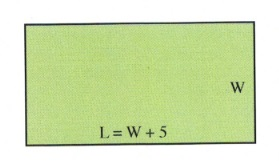Multiplying this out gives us:

$W^2+5W=50$

$W^2+5W-50=0$

Second, we factor this quadratic to get our solution:

$\begin{array}{rrrrrrl} W^2&+&5W&-&50&=&0 \\ (W&-&5)(W&+&10)&=&0 \\ &&&&W&=&5, -10 \\ \end{array}$

We reject the solution $W = -10$.

This means that $L = W + 5 = 5+5= 10$.

Example 10.7.7

If the length of each side of a square is increased by 6, the area is multiplied by 16. Find the length of one side of the original square.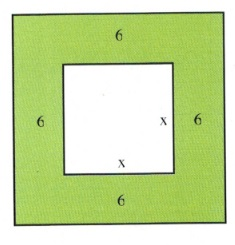The relationship between these two is:

$\begin{array}{rrl} \text{larger area}&=&16\text{ times the smaller area} \\ (x+12)^2&=&16(x)^2 \end{array}$

Simplifying this yields:

$\begin{array}{rrrrrrr} x^2&+&24x&+&144&=&16x^2 \\ -16x^2&&&&&&-16x^2 \\ \hline -15x^2&+&24x&+&144&=&0 \end{array}$

Since this is a problem that requires factoring, it is easiest to use the quadratic equation:

$x=\dfrac{-b\pm \sqrt{b^2-4ac}}{2a},\hspace{0.25in}\text{ where }a=-15, b=24\text{ and }c=144$

Substituting these values in yields $x = 4$ or $x=-2.4$ (reject).

Example 10.7.8

Nick and Chloe want to surround their 60 by 80 cm wedding photo with matting of equal width. The resulting photo and matting is to be covered by a 1 m 2 sheet of expensive archival glass. Find the width of the matting.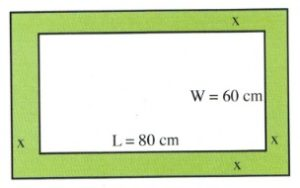$(L+2x)(W+2x)=1\text{ m}^2$

$(80\text{ cm }+2x)(60\text{ cm }+2x)=10,000\text{ cm}^2$

$4800+280x+4x^2=10,000$

$4x^2+280x-5200=0$

Which reduces to:

$x^2 + 70x - 1300 = 0$

Second, we factor this quadratic to get our solution.

It is easiest to use the quadratic equation to find our solutions.

$x=\dfrac{-b\pm \sqrt{b^2-4ac}}{2a},\hspace{0.25in}\text{ where }a=1, b=70\text{ and }c=-1300$

Substituting the values in yields:

$x=\dfrac{-70\pm \sqrt{70^2-4(1)(-1300)}}{2(1)}\hspace{0.5in}x=\dfrac{-70\pm 10\sqrt{101}}{2}$

$x=-35+5\sqrt{101}\hspace{0.75in} x=-35-5\sqrt{101}\text{ (rejected)}$

For Questions 21 to 28, write and solve the equation describing the relationship.

• Find the length and width of a rectangle whose length is 4 cm longer than its width and whose area is 60 cm 2 .
• Find the length and width of a rectangle whose width is 10 cm shorter than its length and whose area is 200 cm 2 .
• A large rectangular garden in a park is 120 m wide and 150 m long. A contractor is called in to add a brick walkway to surround this garden. If the area of the walkway is 2800 m 2 , how wide is the walkway?
• A park swimming pool is 10 m wide and 25 m long. A pool cover is purchased to cover the pool, overlapping all 4 sides by the same width. If the covered area outside the pool is 74 m 2 , how wide is the overlap area?
• In a landscape plan, a rectangular flowerbed is designed to be 4 m longer than it is wide. If 60 m 2 are needed for the plants in the bed, what should the dimensions of the rectangular bed be?
• If the side of a square is increased by 5 units, the area is increased by 4 square units. Find the length of the sides of the original square.
• A rectangular lot is 20 m longer than it is wide and its area is 2400 m 2 . Find the dimensions of the lot.
• The length of a room is 8 m greater than its width. If both the length and the width are increased by 2 m, the area increases by 60 m 2 . Find the dimensions of the room.#### IMAGES

1. Using Quadratic Equations to Solve Problems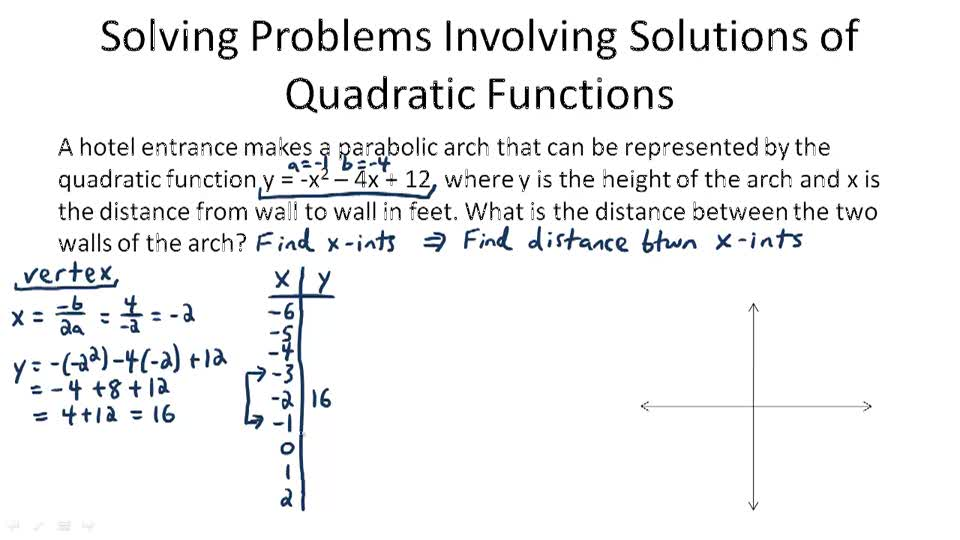2. Using Quadratic Equations to Solve Problems3. Solving Problems involving Quadratic Functions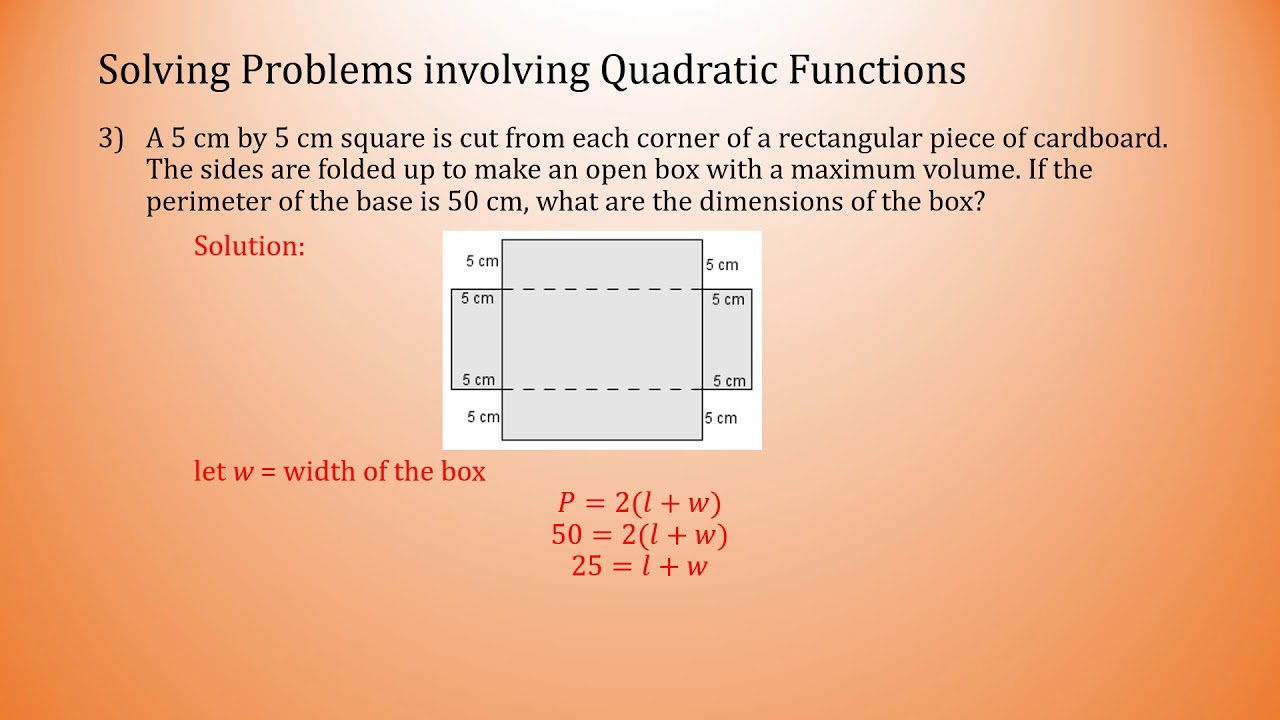4. Solving Problems Involving Quadratic Functions5. Problem solving with quadratic equations questions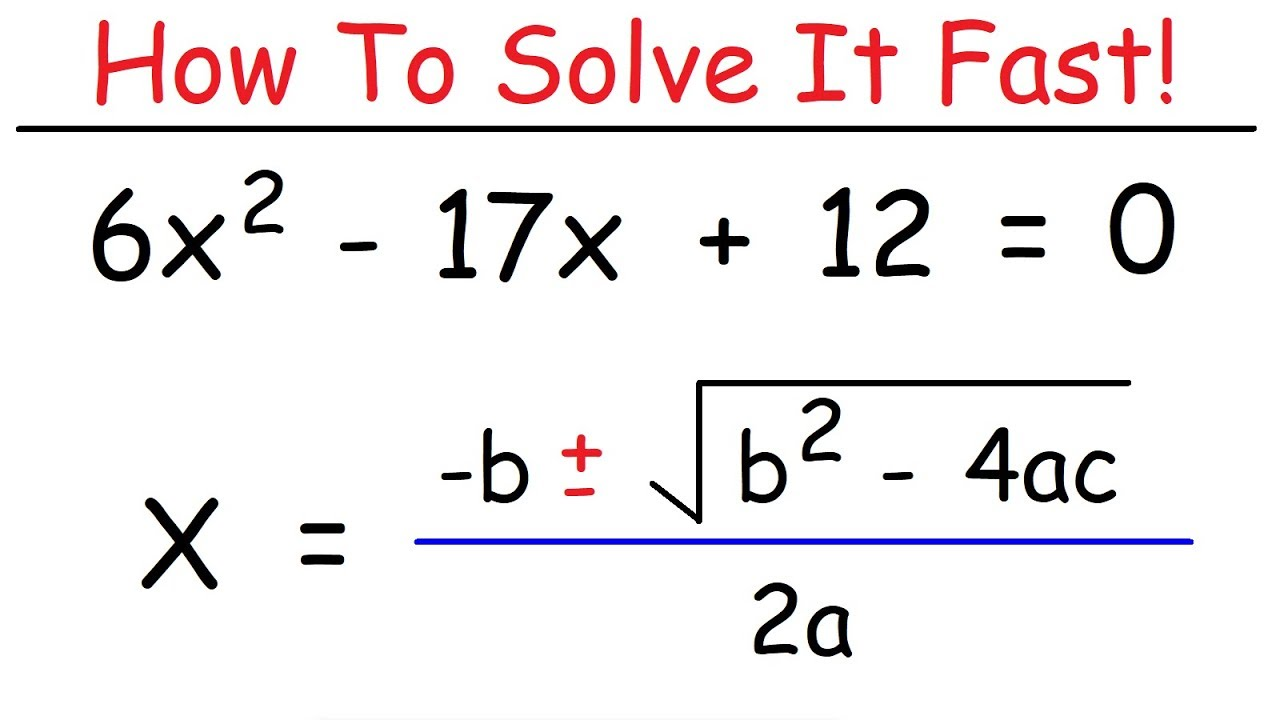#### VIDEO

2. Solving Integer Problems involving Quadratic Equations

3. Video Lesson

4. PROBLEM SOLVING INVOLVING PERMUTATIONS AND COMBINATIONS

1. What Are the Six Steps of Problem Solving?

The six steps of problem solving involve problem definition, problem analysis, developing possible solutions, selecting a solution, implementing the solution and evaluating the outcome. Problem solving models are used to address issues that...

2. How Do You Find the Vertex of a Quadratic Function?

To find the vertex of a quadratic equation, determine the coefficients of the equation, then use the vertex x-coordinate formula to find the value of x at the vertex. Once the x-coordinate is found, plug it into the original equation to fin...

3. Who Created the Polynomials?

There is no one specific person who invented the polynomials, but their history can be traced back to the Babylonians. They used verbal instructions for solving problems related to quadratics.

4. 10.3

Finding the maximum and minimum values of a quadratic function. (10.3.1) – Solve application problems involving quadratic functions. Quadratic equations are

5. Solve problems involving a quadratic function's minimum or

Because the quadratic is not easily factorable in this case, we solve for the intercepts by

6. WORD PROBLEMS INVOLVING QUADRATIC FUNCTIONS PART 1

MATH 9 QUARTER 1 WEEK 10 MELC 16 Solve problems involving quadratic functions FINDING THE EQUATION OF QUADRATIC FUNCTION USING TABLE OF

7. Word Problems Involving Quadratic Equations

You've finally mastered factoring and using the quadratic formula and now you are asked to solve more problems! Except these are even more tough. Now you have

8. 6.7: Applications Involving Quadratic Equations

Just as before, we want to avoid relying on the “guess and check” method for solving applications. Using algebra to solve problems simplifies

Quadratic Function Word Problems ; x, Function, f(x) ; 1, f(x) = 10x + 30, 40 ; -4, f(x) = -2x+1, 9 ; 3, f(x) = x + 50, 53 ; 10, f(x) = 2x, 20

10. Quadratic Functions Problems with Solutions

If D = 0 , the quadratic equation a x 2 + b x + c = 0 has one solution and the graph of f(x) = a x 2 + b x + c has ONE x-intercept. If D > 0 , the quadratic

11. 10.7 Quadratic Word Problems: Age and Numbers

Quadratic-based word problems are the third type of word problems covered in MATQ 1099, with the first being linear equations of one variable and the second

12. How to Solve a Word Problem Involving Optimizing Area by Using a

Step 1: Draw a picture to represent the situation and label the